Processing ......FreeComputerBooks.com Links to Free Computer, Mathematics, Technical Books all over the World

Numerical Analysis and Scientific Computing
Related Book Categories:
 MATLAB Programming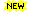Fortran Programming Language Computational and Algorithmic Mathematics Parallel Computing and Programming Computational Simulations and Modeling
•Tea Time Numerical Analysis: Experiences in Mathematics

This textbook was born of a desire to contribute a viable, free, introductory Numerical Analysis textbook. The ultimate goal of this book is to be a complete, one-semester, single-pdf, downloadable textbook designed for mathematics classes.

•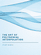The Art of Polynomial Interpolation (Stuart Murphy)

Exploring the techniques of interpolating data allows us to view the development and birth of a polynomial. This book is focused on laying a foundation for understanding and applying several common forms of Polynomial Interpolation.

•Numerical Algorithms: Computer Vision, Machine Learning, etc.

This book presents a new approach to numerical analysis for modern computer scientists, covers a wide range of topics - from numerical linear algebra to optimization and differential equations - focusing on real-world motivation and unifying themes.

•Numerical Methods and MATLAB Programming for Engineers

It covers the fundamentals while emphasizing the most essential numerical methods. Readers will enhance their programming skills using MATLAB to implement algorithms, discover how to use MATLAB to solve problems in science and engineering.

•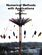Numerical Methods with Applications, 2nd Edition (Autar K Kaw)

This book entitled Numerical Methods with Applications is written primarily for engineering undergraduates taking a course in Numerical Methods. It offers a unique treatise to numerical methods which is based on a holistic approach and short chapters.

•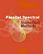Parallel Spectral Numerical Methods (Gong Chen, et al)

This book teaches the principals of Fourier spectral methods, their utility in solving partial differential equation and how to implement them in code. The programs will use Matlab and Fortran. A Python implementation of some of the Matlab programs is also provided.

•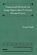Numerical Methods for Large Eigenvalue Problems (Yousef Saad)

This book is intended for researchers in applied mathematics and scientific computing as well as for practitioners interested in understanding the theory of numerical methods used for eigenvalue problems.

•First Semester in Numerical Analysis with Julia (Giray Okten)

This book presents the theory and methods, together with the implementation of the algorithms using the Julia programming language. The book covers computer arithmetic, root-finding, numerical quadrature and differentiation, and approximation theory.

•Numerical Analysis - Theory and Application (Jan Awrejcewicz)

This book is a book focused on introducing theoretical approaches of numerical analysis as well as applications of various numerical methods to either study or solving numerous theoretical and engineering problems.

•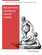High Performance Computing and Numerical Modelling

This textbook provides a step-by-step approach to numerical methods in engineering modelling. It provides a consistent treatment of the topic, from the ground up, to reinforce for students that numerical methods are a set of mathematical modelling tools.

•Numerical Computing with MATLAB (Cleve B. Moler)

This book is a lively textbook for an introductory course in numerical methods, MATLAB, and technical computing. It helps readers learn enough about the mathematical functions in MATLAB to use them correctly, and modify them appropriately, etc.

•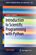Introduction to Scientific Programming with Python

This book offers an initial introduction to programming for scientific and computational applications using the Python programming language. The presentation style is compact and example-based, assuming little or no prior experience in programming.

•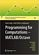Programming for Computations - MATLAB/Octave (Svein Linge)

This book presents computer programming as a key method for solving mathematical problems using MATLAB and Octave. It is intended for novice programmers. Each treated concept is illustrated and explained in detail by means of working examples.

•Programming for Computations - Python 3.6 Edition

This book outlines the shortest possible path from no previous experience with programming to a set of skills that allows students to write simple programs for solving common mathematical problems with numerical methods in the context.

•Introduction to GNU Octave (Jason Lachniet)

This is a practical, step-by-step guide that will help you to quickly become a proficient GNU Octave user. The book is packed with clear examples, screenshots, and code to carry out your data analysis without any problems.

•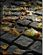Introduction to High-Performance Scientific Computing (Eijkhout)

This is a textbook that teaches the bridging topics between numerical analysis, parallel computing, code performance, large scale applications. it assumes a basic knowledge of numerical computation and proficiency in Fortran or C programming.

•Scientific Programming and Computer Architecture

The book digs into linkers, compilers, operating systems, and computer architecture to understand how the different parts of the computer interact with programs. It begins with a review of C/C++ and explanations of how libraries, linkers, and Makefiles work.

•Financial Numerical Recipes in C++: Applications in Finance

This book provides a good deal of useful examples and algorithms for people working within the field of finance, in C++. All the routines have been made to confirm to the new ISO/ANSI C++ standard, using namespaces and the standard template library.

•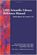GNU Scientific Library Reference Manual (Brian Goug, et al)

The GNU Scientific Library (GSL) is a free numerical library for C and C++ programmers. It provides over 1,000 routines for solving mathematical problems in science and engineering. This reference manual is the definitive guide to the library.

•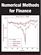Numerical Methods for Finance (Robert Nurnberg)

This book explores new and relevant numerical methods for the solution of practical problems in finance. It is one of the few books entirely devoted to numerical methods as applied to the financial field.

•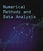Fundamental Numerical Methods and Data Analysis (G. W. Collins)

The basic premise of this book is that it can serve as the basis for a wide range of courses that discuss numerical methods used in data analysis and science. To reflect this, the subject matter is wide ranging and perhaps too broad for a single course.

•Finite Difference Computing with PDEs: A Software Approach

This easy-to-read book introduces the basics of solving partial differential equations by means of finite difference methods. Unlike many of the traditional academic works on the topic, this book was written for practitioners.

•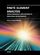Finite Element Analysis: Biomedical Apps to Industrial

This book presents represents a numerical technique for finding approximate solutions to partial differential equations as well as integral equations, permitting the numerical analysis of complex structures based on their material properties.

•Finite Element Analysis (David Moratal)

This book provides a bird's eye view on this very broad matter through original and innovative research studies exhibiting various investigation directions. You will gain a better understanding of where Finite Element Analysis stands today.

•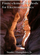Finite-element Methods for Electromagnetics (Stanley Humphries)

This book covers a broad range of practical applications involving electric and magnetic fields. The text emphasizes finite-element techniques to solve real-world problems in research and industry.

•Programming for Computations - Python (Svein Linge, et al)

This book presents computer programming as a key method for solving mathematical problems using Python. Each treated concept is illustrated and explained in detail by means of working examples. It is intended for novice programmers and engineers.

•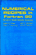Numerical Recipes in Fortran 90: Art of Parallel Scientific Computing

This book contains a detailed introduction to the Fortran 90 language and to the basic concepts of parallel programming, plus source code for all routines from the second edition of Numerical Recipes.

•Numerical Recipes in Fortran 77: The Art of Scientific Computing

The product of a unique collaboration among four leading scientists in academic research and industry, Numerical Recipes is a complete text and reference book on scientific computing.

•Numerical Recipes in C: The Art of Scientific Computing

Numerical Recipes is a complete text and reference book on scientific computing. This is the second edition with C programming.

•Solving PDEs in Python: The FEniCS Tutorial I (H. Langtangen)

This book offers a concise and gentle introduction to finite element programming in Python based on the popular FEniCS software library. Using a series of examples, it guides readers through the essential steps to quickly solving a PDE in FEniCS.

•LAPACK95 Users' Guide (Vincent Barker, et al)

This book provides an introduction to the design of the LAPACK95 package, a detailed description of its contents, reference manuals for the leading comments of the routines, and example programs.

•LAPACK Users' Guide, 3rd Edition (E. Anderson, Z. Bai, et al)

This free book provides an introduction to the design of the LAPACK package, a detailed description of its contents, reference manuals for the leading comments of the routines, and example programs

•Introduction to Fortran 95 and Numerical Computing (Adrian Sandu)

This book provides an introduction to its latest standard: Fortran 95, the most important language for scientific and engineering computation.

•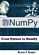From Python to NumPy (Nicolas P. Rougier)

NumPy is one of the most important scientific computing libraries available for Python. This book teaches you how to achieve expert level competency to perform complex operations, with in-depth coverage of advanced concepts.

•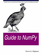Guide to NumPy (Travis E. Oliphant)

This book is for programmers, scientists, or engineers, who have basic Python knowledge and would like to be able to do numerical computations with Python. It will give you a solid foundation in NumPy arrays and universal functions.

•NumPy Tutorials (Usman Malik, Anne Bonner, et al)

They provide everything you need to know to get started with NumPy. They also explain the basics of NumPy such as its architecture and environment, discusses the various array functions, types of indexing, etc. With examples for better understanding.

•Scipy Lecture Notes (Emmanuelle Gouillart, et al)

This book is the teaching material on the scientific Python ecosystem, a quick introduction to central tools and techniques. It is for programmers from beginner to expert. Work on real-world problems with SciPy, NumPy, Pandas, scikit-image, and other Python libraries.

•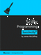SciPy Programming Succinctly (James McCaffrey)

This book offers readers a quick, thorough grounding in knowledge of the Python open source extension SciPy. The SciPy library, accompanied by its interdependent NumPy, offers Python programmers advanced functions that work with arrays and matrices.

•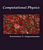Computational Physics and Scientific Computing: C++ or Fortran

This book is an introduction to the computational methods used in physics, but also in other scientific fields. Both C++ or Fortran are used for programming the core programs and data analysis is performed using the powerful tools of the Gnu/Linux environment.

•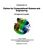Introduction to Python for Computational Science and Engineering

This book summarises a number of core ideas relevant to Computational Engineering and Scientific Computing using Python. The emphasis is on introducing some basic Python (programming) concepts that are relevant for numerical algorithms.

•Scientific Computing with MATLAB (Paul Gribble)

The goal of this book is to provide you with skills in scientific computing: tools and techniques that you can use in your own scientific research. We will focus on learning to think about experiments and data in a computational framework.

•Numerical Simulations of Physical and Engineering Processes

The book handles the numerical simulations of physical and engineering systems. It can be treated as a bridge linking various numerical approaches of two closely inter-related branches of science, i.e. physics and engineering.

•Applied Computational Fluid Dynamics (Hyoung Woo Oh)

This book is served as a reference text to meet the needs of advanced scientists and research engineers who seek for their own computational fluid dynamics (CFD) skills to solve a variety of fluid flow problems.

Book Categories
 :All CategoriesRecent BooksMiscellaneous BooksComputer LanguagesComputer ScienceData Science/DatabasesElectrical EngineeringJava and Java EE (J2EE)Linux and UnixMathematicsMicrosoft and .NETMobile ComputingNetworking and CommunicationsSoftware EngineeringSpecial TopicsWeb Programming
Other Categories